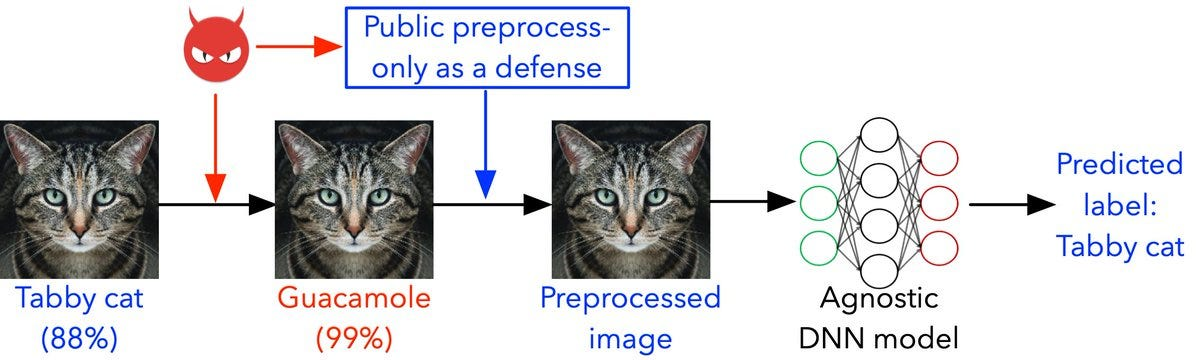# Threat of Adversarial Attacks on Deep Learning in Computer Vision# Threat of Adversarial Attacks on Deep Learning in Computer Vision

A step towards comprehensive understanding of Adversarial Attack

Research showed that despite high accuracies of neural networks, modern deep networks are susceptible to adversarial attacks in form of small perturbations to images that remain almost imperceptible to human vision system.Such attacks can cause a neural network classifier to completely change it’s prediction about image. Even worst, the attacked model even report with high confidence on wrong prediction.Moreover the same image can fool multiple network classifiers.

Let’s first understand some terminologies:

1.Adversarial Example/Image: Is modified version of clean image that is intentionally perturbed to fool a machine learning technique, such as neural networks.

2. Adversarial perturbation: Is noise that is added to the clean image to make it adversarial example.

4.Black box attacks:feed a targeted model with adversarial examples that are generated without knowledge of that model.

5.White box attacks: attacks assume complete knowledge of the targeted model, including parameter values, architecture, training method training data as well

7.One shot/ One step methods: generate adversarial perturbation by performing a single step computation.

8.Transferability: refers to ability of of adversarial example to remain effective even for models other than one used to generate it.

9.Targeted Attack: The adversary might want to generate attack samples that causes false classification to any other class than correct correct i.e. un targeted attack or can produce samples that forces the model to predict a specific target class.

# Attacks for Classification

This is a white box attack whose goal is to ensure misclassification. A white box attack is where the attacker has complete access to the model being attacked. One of the most famous examples of an adversarial image shown below is taken from the aforementioned paper.

Here, starting with the image of a panda, the attacker adds small perturbations (distortions) to the original image, which results in the model labelling this image as a gibbon, with high confidence. The process of adding these perturbations is explained below.

The fast gradient sign method works by using the gradients of the neural network to create an adversarial example.

FGSM perturbs an image to increase loss of classifier on resulting image.

`perturbation = epsilon * sign(delta J(theta, Ic, l))Adversarial image = original image + perturbation.`

delta J computes the gradient of the cost function around current value of model parameters wrt. parameters theta wrt. Ic, sign() denotes sign function and c is small value to restrict norm of perturbation.

Intrestingly, the adversarial examples generated by FGSM exploit linearity of Deep Neural Networks.

## 2. ONE STEP TARGET CLASS VARIATION OF FGSM

Instead of using true label l of an image, they used label l(target) of least likely predicted class predicted by network for Ic. The computed perturbation is then substracted from the original image to make it adversarial example. For NN with cross entropy loss doing so maximizes the probability that the network predicts l(target) as label for adversarial example.

Let’s load the pre trained MobileNetV2 model and the ImageNet class names. Model predicted the image as Labrador_retriever with 41.82% confidence.

Result after Attack:

3. Jacobian-Based Saliency Map Attack — Targeted Fooling

JSMA is another gradient based whitebox method. Papernot et al. (2016) proposed to use the gradient of loss with each class labels with respect to every component of the input i.e. jacobian matrix to extract the sensitivity direction. Then a saliency map is used to select the dimension which produces the maximum error using the following equation:

Let’s again try to create adversarial inputs that will fool our network to classify digit 2 as 6, but this time with as little perturbations as possible.

1. Algorithm modifies pixels of clean image one at a time and moniters the effect of change on resulting classification.

2. This monitering is performed by computing saliency map using the gradients of the output of the network layers

3. In this map, a larger value indicates a higher likelihood of fooling network to predict l(target) as label of modified image instead of original label l.

4. Once map is computed, algorithm chooses the pixel that is most effective to fool netwrok and alters it.

5. This process is repeated until either the max no. of allowable pixels are altered.

Output:

4. Carlini and Wagner Attacks

5. Deep Fool